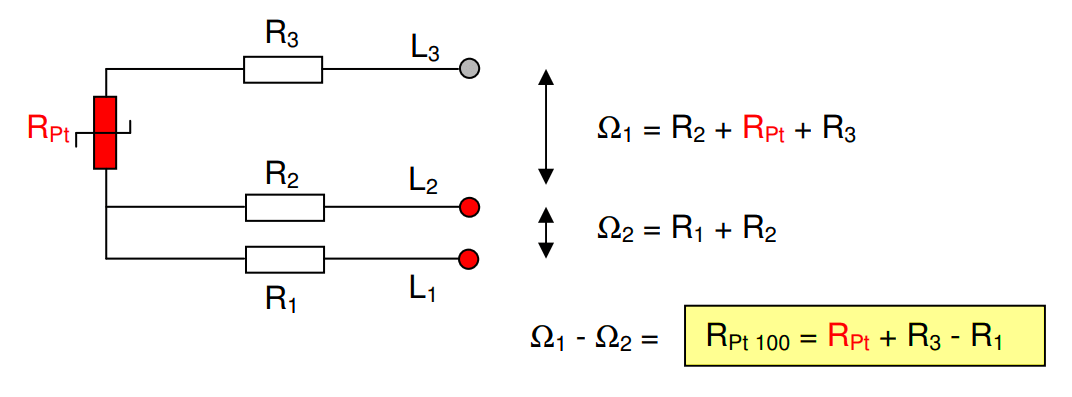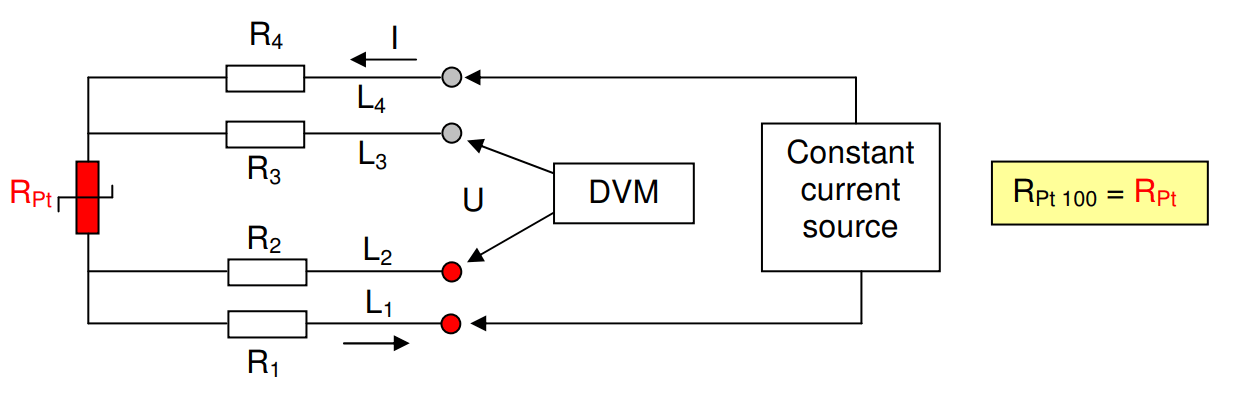# Formula of 2 Wire RTD, 3 Wire RTD & 4 Wire RTD

## 2 Wire RTD :where

• Rpt is Resistance of RTD
• R2 is Resistance of first lead wire (Extension cable used to connect RTD)
• R3 is Resistance of second lead wire (Extension cable used to connect RTD)
• L2 & L3 are extension cable leads

The wire resistances R2 and R3 are inevitably included in the measured value. Wire resistance can be cancelled by calibration, but just at one specific temperature of the wire (often room temperature).

Also Read :  Difference Between 2 wire RTD, 3 wire RTD, & 4 wire RTD

## 3 Wire RTD :where

• Rpt is Resistance of RTD
• R1 is Resistance of first lead wire (Extension cable used to connect RTD)
• R2 is Resistance of second lead wire (Extension cable used to connect RTD)
• R3 is Resistance of third lead wire (Extension cable used to connect RTD)
• L1, L2 & L3 are extension cable leads
• Ω1 is the total resistance between lead wires L2 & L3
• Ω2 is the total resistance between lead wires L1 & L2

If and only if the wire resistances R1 and R3 are equal you measure the true Pt100 resistance. As the wire L2 also is coloured red (and hard to follow) you have to keep all the wire resistances R1, R2 and R3 equal.

Also Read : Difference Between RTD, Thermocouple & Thermistor

## 4 Wire RTD :The current source will maintain the excitation current constantly (0.1 – 1 mA). Following the Law of Ohm, R = U / I, the resistance can be calculated. The digital volt meter, DVM, has an input impedance of at least 10 Mohms.

Thus just an extremely small current will pass through the DVM which means that the voltage drops over the wire resistances R2 and R3 will be practically none.

Source : pentronic.se